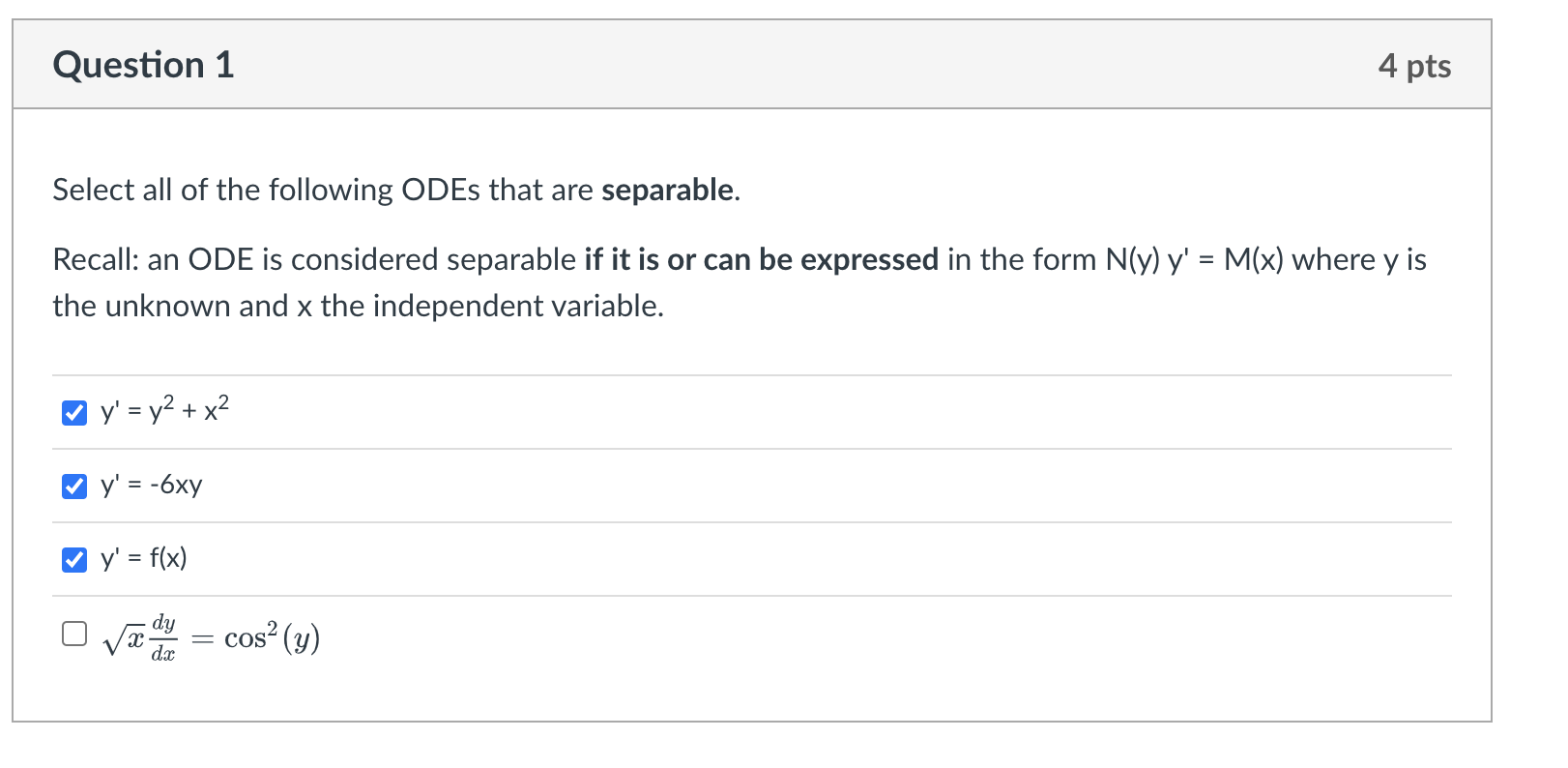Home / Expert Answers / Calculus / explain-why-and-how-select-all-of-the-following-odes-that-are-separable-recall-an-ode-is-consider-pa350

# (Solved): explain why and how Select all of the following ODEs that are separable. Recall: an ODE is consider ...explain why and how

Select all of the following ODEs that are separable. Recall: an ODE is considered separable if it is or can be expressed in the form $$N(y) y^{\prime}=M(x)$$ where $$y$$ is the unknown and $$x$$ the independent variable. $y^{\prime}=y^{2}+x^{2}$ $y^{\prime}=-6 x y$ $y^{\prime}=f(x)$ $\sqrt{x} \frac{d y}{d x}=\cos ^{2}(y)$

We have an Answer from Expert